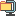검색어 입력폼

# DSP 매트랩 #1 나이키스트 이론

저작시기 2009.05 |등록일 2009.05.31압축파일 (zip) | 7페이지 | 가격 2,000원

## 소개글

Homework (Matlab#1)

1. Spectrum of a signal is as follows;

(a) Determine from its spectrum.
(b) Write a matlab function `DiscreteSignal` that produces discretized version of . The matlab file "DiscreteSignal.m" is as following;

function [xn,t]=DiscreteSignal(Ts,TimeRange);
% Ts: sampling time interval
% TimeRange : Observation time
% xn : discrete signal xn=x(n*Ts)
% t : sampling points at time index, that is, "t=0:Ts:TimeRange"

(c) Determine Nyquist sampling time Ts, and discretize x(t) with the Ts. Overlay plots of the discrete signals by using `stem(t,xn)` and `plot(t,xn)`.

(d) Sample x(t) with the Ts/2. Overlay plots of the discrete signals by using `stem(t,xn)` and `plot(t,xn)`.

(e) Sample x(t) with the Ts/5. Overlay plots of the discrete signals by using `stem(t,xn)` and `plot(t,xn)`.

(f) Observe discrete signals as varying sampling time.

(g) Discretize x(t) with the Ts/2. Plot the discrete signal by using `stem(xn)`.

(h) Note the difference between `stem(t,xn)` and `stem(xn)`.

* Things to be turned in.
- Source code

## 목차

1. Spectrum of a signal is as follows;
(a) Determine from its spectrum.
(b) Write a matlab function `DiscreteSignal` that produces discretized version of .
(c) Determine Nyquist sampling time Ts, and discretize x(t) with the Ts. Overlay plots of the discrete signals by using `stem(t,xn)` and `plot(t,xn)`.

## 본문내용

1. Spectrum of a signal is as follows;

(a) Determine from its spectrum.
x(t) = 10cos(40pi + 3.2pi) + 5cos(70pi) + 2.5cos(130pi-0.2pi)

>> F0=5; %fundamental freq
Init_t=-0.2; %초기시간
ts=0.001; %시간간격
Final_t=0.2; %최종시간
t=Init_t:ts:Final_t; %시간영역설정
xt=10*cos(2*4*F0*pi*t+3.2*pi)+5*cos(2*7*F0*pi*t)+2.5*cos(2*13*F0*pi*t-0.2*pi);
%xt 함수 정의.
plot(t,xt); %xt 에 대한 그래프

분석
fundamental frequency를 5로 정의하고 -0.2초부터 2초까지의 signal을 0.001초의 샘플링타임으로 그래프 파형을 그렸다. 약 0.1초의 주기를 확인할 수 있으며 cosine 세 개의 그래프가 더해진 형태의 파형을 확인할 수 있다.

(b) Write a matlab function `DiscreteSignal` that produces discretized version of . The matlab file "DiscreteSignal.m" is as following;

function [xn,t]=DiscreteSignal(Ts,TimeRange);
% Ts: sampling time interval
% TimeRange : Observation time
% xn : discrete signal xn=x(n*Ts)
% t : sampling points at time index, that is, "t=0:Ts:TimeRange"

%DiscreteSignal.m
function [xn,t]=DiscreteSignal(Ts,TimeRange);

없음

(b)그래프 0.001.JPG
(b)그래프.JPG
(c)그래프1.JPG
(c)그래프2.JPG
(c)그래프3.JPG
(d)그래프.JPG
(e)그래프.JPG
(g)stem1.jpg
(g)stem2.jpg
1.jpg
5.jpg
10.jpg
a5.jpg
a10.jpg
a100.jpg
a1000.jpg
Matlab(HW1).hwp
Matlab-HW1문제.hwp
(a)그래프.JPG